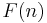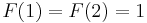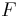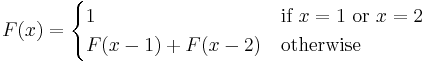# Recursive function

(diff) ← Older revision | Latest revision (diff) | Newer revision → (diff)
Let$F(n)$ denote the$n$th Fibonacci number, where$n$ is a natural number and$F(1) = F(2) = 1$. We may define$F$ as a function, as follows:$F(x) = \begin{cases} 1 & \text{if }x = 1\text{ or }x = 2 \\ F(x-1) + F(x-2) & \text{otherwise} \end{cases}$